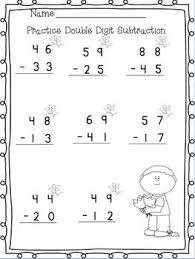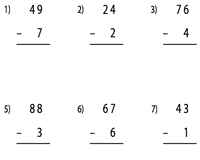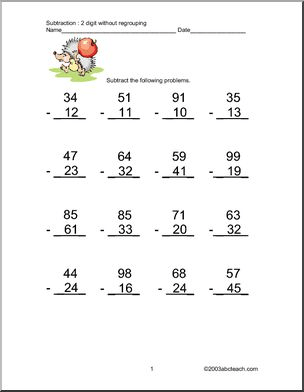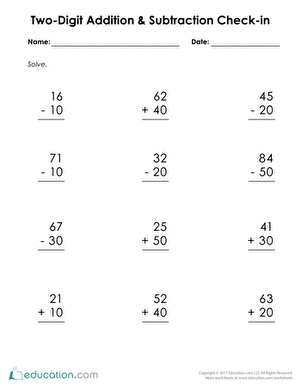# SUBTRACTION OF 2-DIGIT NUMBERS WITHOUT REGROUPING

HELLO CHILDREN, WELCOME BACK TO CLASS

Subtracting means taking away one number from another number. Usually a bigger number from a smaller number. To subtract 2- digit numbers from themselves,

Place one number above the other so the tens’ place digits and ones’ place digits are lined up. Draw a line under the bottom number.

6     7   =    60   +    7

–   4     1       – 40   +    1

————-

Subtract the bottom ones’ place digit (1) from the top ones’ place digit (7) and place the difference (6) below the line in the ones’ place column.

6       7

–  4      1

6

Subtract the bottom tens’ place digit (4) from the top tens’ place digit (6). Place the difference (2) below the line in the tens’ place column.

6     7        =   60   +   7

–   4     1           – 40   +   1

—————

2    6              20   +   6ACTIVITY

• Arrange and Solve

1. 57 – 16
2. 64 – 43
3. 95 – 42
4. 88 – 44
5. 59 – 27
6. 48 – 31
7. 86 – 46
8. 93 – 81Your Opinion Matters! Quickly tell us how to improve your Learning Experience HERE